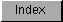```
The IEEE single-precision transcendental math library was introduced in
V36.  It contains entries for transcendental math functions such as sine,
cosine, and square root.

This library resides on disk and is opened by calling OpenLibrary() with
"mathieeesingtrans.library" as the argument.  Do not share the library
base pointer between tasks -- see note at beginning of chapter.

#include <exec/types.h>
#include <libraries/mathieeesp.h>

struct Library *MathIeeeSingTransBase;

#include <clib/mathsingtrans_protos.h>

VOID main()
{
if (MathIeeeSingTransBase = OpenLibrary("mathieeesingtrans.library",37))
{
. . .

CloseLibrary(MathIeeeSingTransBase);
}
else  printf("Can't open mathieeesingtrans.library\n");
}

The global variable MathIeeeSingTransBase is used internally for all
future library references.

The IEEE single-precision transcendental math library is dependent upon
the IEEE single-precision basic math library, which it will open if it is
not open already.  If you want to use the IEEE single-precision basic math
functions in conjunction with the transcendental math functions however,
you have to specifically open the basic math library yourself.

Just as the IEEE single-precision basic math library, the IEEE
single-precision transcendental math library will take advantage of a
680X0/68881 combination or another math resource, if present.

SP IEEE Transcendental Functions (V36 Or Greater)

```